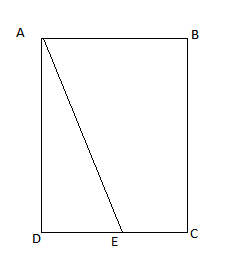Rectangle reflections

Geometry Level 3In a rectangle $ABCD$ a point $E$ is taken on $CD$ such that the reflection of $D$ from the line mirror $AE$ lies on $BC$

Given $AB=7 \text m$, $BC=25 \text m$ find distance $DE$.

×